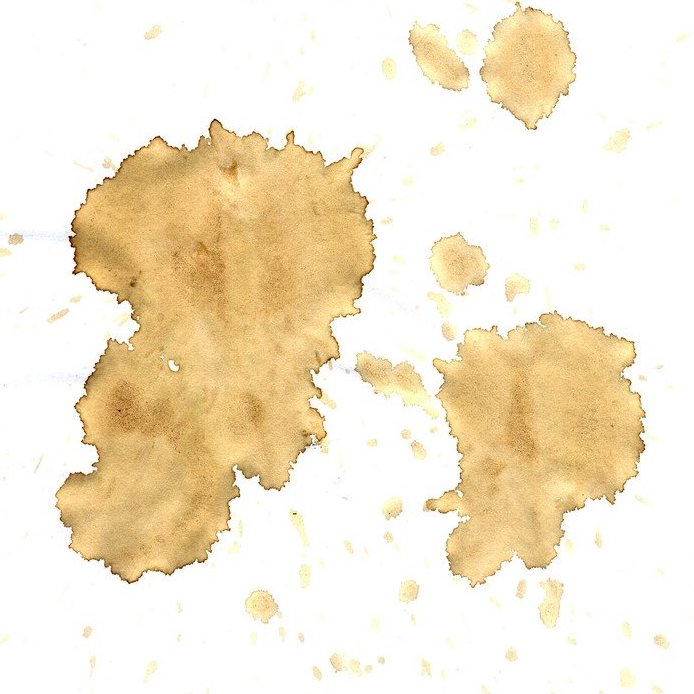# How much darker is the edge of a coffee stain than its center?

A comathematician is a device for turning cotheorems into ffee.
–the dual of Rényi’s famous jokeLeft: photograph of a coffee stain, showing a thin dark ring around a lighter interior. Right: DALL-E also knows that the edge of a coffee spill becomes darker than the center, even if the spill is in the shape of a face.

We simplify the coffee stain appearance into two regions: a ring and a uniform interior region. Suppose the puddle is a circle of radius $$R$$ and the ring has width $$\delta r$$. For notational simplicity, we shall normalize the evaporation time to $$t \in [0, 1]$$. Let $$m_R(t)$$ be the mass of coffee in the ring region at time $$t$$. The stain occurs by coffee soaking into the, say, white tablecloth; if the coffee is more concentrated, more coffee particles will soak into the surface. The rate of the mass of coffee soaking through the surface at some position is thus proportional to the mass of solute in the coffee solution directly above that position. Integrating over time to count all the coffee that has soaked into the tablecloth, the ratio $$C$$ of the mass of coffee in the ring region to the mass of coffee within the ring is \begin{aligned} C \sim \frac{\int_0^1 \frac{m_R(t)}{2\pi R\delta r}dt}{\int_0^1 \frac{m_0-m_R(t)}{\pi r^2}dt} \sim \frac{1}{2}\frac{R}{\delta r} \frac{\int_0^1 m_R(t)~dt}{\int_0^1 m_0-m_R(t)~dt}, \end{aligned} where $$m_0$$ is the total mass of solute in the puddle. We can estimate $$m_R(t)$$ from a basic understanding of the mechanism behind the ring formation. At the edge of the puddle, the contact angle — i.e., the angle between the liquid-gas interface and the liquid-solid interface — is primarily a function of material properties (given by Young’s equation), not the size of the puddle. As the puddle evaporates, the water attempts to maintain this angle to successfully minimize free energy. However, due to the roughness of the liquid-solid interface, reducing the puddle radius would require the contact angle to drastically change. This energy barrier against shrinking the puddle effectively pins down the edge of the puddle despite evaporation. As the puddle evaporates, coffee from the interior must flow outwards to the edge to maintain the contact angle.

Consider coffee at distance $$r$$ from the center of the puddle. By symmetry, when a coffee particle initially at position $$r$$ eventually flows to the edge of puddle, all coffee particles at radii larger than $$r$$ have already arrived at the edge. That is, $$m_R$$ is proportional to $$R^2 - r^2$$. But when does the particle that started at $$r$$ ultimately arrive at the edge? The rate of evaporation is approximately constant over time. To maintain the contact angle, the volume of water that must arrive at the edge per unit time is time-independent. As time proceeds, there is less and less water in the interior to flow out. Consequently, the flow velocity must increase over time: a point starting at $$r \approx R$$ will arrive at time $$t \approx 0$$ with small velocity, and a point starting at $$r \approx 0$$ will arrive at time $$t \approx 1$$ with large velocity. To lowest order, we see that $$r \sim R(1 - t^2)$$ satisfies these boundary conditions. Combining these two observations, we conclude that \begin{aligned} m_R(t) \sim R^2 - R^2(1-t^2)^2 \sim R^2 t^4 \end{aligned} up to a proportionality constant given by the density of solute. Similarly dropping the density from $$m_0$$, we have \begin{aligned} C \sim \frac{1}{2}\frac{R}{\delta r} \frac{\int_0^1 t^4}{1 - t^4} \sim \frac{1}{8}\frac{R}{\delta r}. \end{aligned} Since the opacity of the ring is given by the probability of scattering off electrons in the coffee particles, increasing the density linearly decreases the opacity: the ratio of the opacity in the ring to the opacity in the interior is $$8\delta r / R$$. So a puddle has radius $$R \sim 5$$ cm, but what is the width $$\delta r$$ of the ring?

As described by our ring formation mechanism, surface tension pulls particles coffee towards the edge of the puddle to keep the contact angle fixed over time. Once the edge of the ring is saturated by coffee particles, new particles will arrive at a smaller radius, causing the ring to grow. For a contact angle $$\theta$$, the ring will fill with solid mass at width $$\delta r$$ satisfying \begin{aligned} \frac{1}{2} \cdot 2\pi R \delta r \cdot \delta r \tan\theta &= \frac{R/8\delta r}{1 + R/8\delta r} \sim 1 \end{aligned} since $$\delta r \ll R$$. Evaluating \begin{aligned} \delta r \sim \frac{1}{R}\frac{1}{\sqrt{\pi \tan\theta}} \end{aligned} for $$\theta \sim 45^\circ$$ (since a tablecloth isn’t hydrophobic) gives $$\delta r \sim 0.1$$ cm. Plugging into the opacity ratio $$8\delta r/R$$, we conclude that the interior of the stain is 15% the darkness of the ring. Note that the estimate is relatively independent of $$\theta$$ (to order of magnitude): choosing $$\theta = 15^\circ$$ increases the opacity by a factor of two, and $$\theta = 80^\circ$$ reduces the opacity by a factor of two.

## References

The seminal papers that really got the field of coffee stains going are [1,2], which have almost 10,000 citations in total. (They show the underlying mechanism of pinning the contact line and the consequent flow of material due to surface tension, but the rest of their analysis focuses on solving differential equations either numerically or in limiting regimes.) Also, for more on contact angles and Young’s equation, we highly recommend the Wikipedia page entitled “Wetting.”

 Deegan, Robert D., et al. “Capillary flow as the cause of ring stains from dried liquid drops.” Nature 389.6653 (1997): 827-829.
 Deegan, Robert D., et al. “Contact line deposits in an evaporating drop.” Physical review E 62.1 (2000): 756.